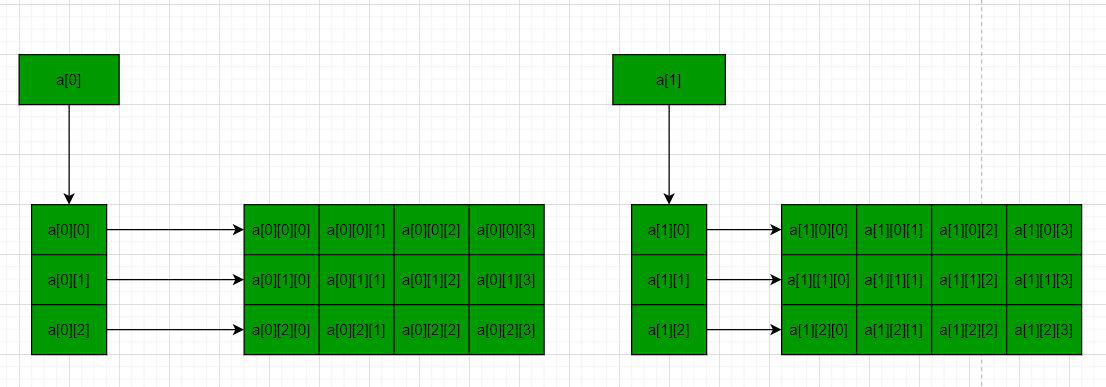# How to dynamically allocate a 3D array in C++

Prerequisite: Array Basics
In C/C++, multidimensional arrays in simple words as an array of arrays. Data in multidimensional arrays are stored in tabular form (in row major order). Below is the general form of declaring N-dimensional arrays:

Syntax of a Multidimensional Array:

data_type  array_name[size1][size2]….[sizeN];

data_type: Type of data to be stored in the array.
Here data_type is valid C/C++ data type
array_name: Name of the array
size1, size2, …, sizeN: Sizes of the dimensions

3-D arrays are an array of Double dimensional arrays:

Syntax of a 3D array:

data_type array_name[x][y][z];
data_type: Type of data to be stored. Valid C/C++ data type.

For more details on multidimensional and, 3D arrays, please refer to the Multidimensional Arrays in C++ article.

Problem: Given a 3D array, the task is to dynamically allocate memory for a 3D array using new in C++.

Solution: In the following methods, the approach used is to make two 2-D arrays and each 2-D array is having 3 rows and 4 columns with the following values.

```1 2 3 4
5 6 7 8
9 10 11 12

13 14 15 16
17 18 19 20
21 22 23 24```

X = No of 2D arrays.
Y = No of rows of each 2D array.
Z = No of columns of each 2D array.

Method 1: using single pointer – In this method, a memory block of size x*y*z is allocated and then the memory blocks are accessed using pointer arithmetic. Below is the program for the same:

## C++

 `// C++ program to dynamically allocate` `// the memory for 3D array in C++` `// using new operator` `#include ` `using` `namespace` `std;`   `// Driver Code` `int` `main()` `{` `    ``// Dimensions of the 3D array` `    ``int` `x = 2, y = 3, z = 4;` `    ``int` `count = 0;`   `    ``// Allocate memory blocks` `    ``// of size x*y*z` `    ``int``* a = ``new` `int``[x * y * z];`   `    ``// Traverse the 3D array` `    ``for` `(``int` `i = 0; i < x; i++) {` `        ``for` `(``int` `j = 0; j < y; j++) {` `            ``for` `(``int` `k = 0; k < z; k++) {`   `                ``// Assign values to the` `                ``// memory blocks created` `                ``*(a + i * y * z + j * z + k) = ++count;` `            ``}` `        ``}` `    ``}`   `    ``// Traverse the 3D array again` `    ``for` `(``int` `i = 0; i < x; i++) {` `        ``for` `(``int` `j = 0; j < y; j++) {` `            ``for` `(``int` `k = 0; k < z; k++) {`   `                ``// Print values of the` `                ``// memory blocks created` `                ``cout << *(a + i * y * z + j * z + k) << ``" "``;` `            ``}` `            ``cout << endl;` `        ``}` `        ``cout << endl;` `    ``}`   `    ``// Deallocate memory` `    ``delete``[] a;`   `    ``return` `0;` `}`

Output:

```1 2 3 4
5 6 7 8
9 10 11 12

13 14 15 16
17 18 19 20
21 22 23 24```

Method 2: using triple pointer – Below is the diagram to illustrate the concept:Below is the program for the same:

## C++

 `// C++ program to dynamically allocate` `// the memory for 3D array in C++` `// using new operator` `#include ` `using` `namespace` `std;`   `// Driver Code` `int` `main()` `{` `    `  `    ``// Dimensions of the 3D array` `    ``int` `x = 2, y = 3, z = 4;` `    ``int` `count = 0;`   `    ``// Allocate memory blocks of size` `    ``// x i.e., no of 2D Arrays` `    ``int``*** a = ``new` `int``**[x];`   `    ``for` `(``int` `i = 0; i < x; i++) {`   `        ``// Allocate memory blocks for` `        ``// rows of each 2D array` `        ``a[i] = ``new` `int``*[y];`   `        ``for` `(``int` `j = 0; j < y; j++) {`   `            ``// Allocate memory blocks for` `            ``// columns of each 2D array` `            ``a[i][j] = ``new` `int``[z];` `        ``}` `    ``}`   `    ``for` `(``int` `i = 0; i < x; i++) {` `        ``for` `(``int` `j = 0; j < y; j++) {` `            ``for` `(``int` `k = 0; k < z; k++) {`   `                ``// Assign values to the` `                ``// memory blocks created` `                ``a[i][j][k] = ++count;` `            ``}` `        ``}` `    ``}`   `    ``for` `(``int` `i = 0; i < x; i++) {` `        ``for` `(``int` `j = 0; j < y; j++) {` `            ``for` `(``int` `k = 0; k < z; k++) {`   `                ``// Print values of the` `                ``// memory blocks created` `                ``cout << a[i][j][k] << ``" "``;` `            ``}` `            ``cout << endl;` `        ``}` `        ``cout << endl;` `    ``}`   `    ``// Deallocate memory` `    ``for` `(``int` `i = 0; i < x; i++) {` `        ``for` `(``int` `j = 0; j < y; j++) {` `            ``delete``[] a[i][j];` `        ``}` `        ``delete``[] a[i];` `    ``}` `    ``delete``[] a;`   `    ``return` `0;` `}`

Output:

```1 2 3 4
5 6 7 8
9 10 11 12

13 14 15 16
17 18 19 20
21 22 23 24```

Feeling lost in the world of random DSA topics, wasting time without progress? It's time for a change! Join our DSA course, where we'll guide you on an exciting journey to master DSA efficiently and on schedule.
Ready to dive in? Explore our Free Demo Content and join our DSA course, trusted by over 100,000 geeks!

Previous
Next